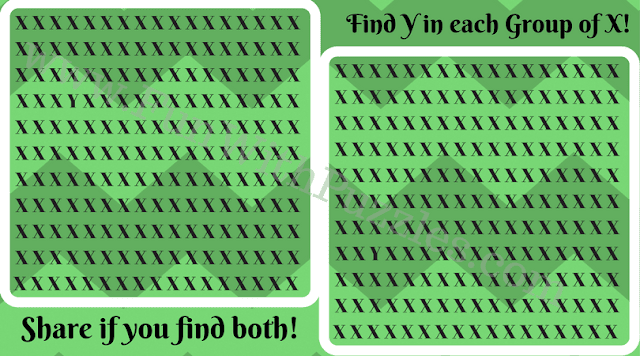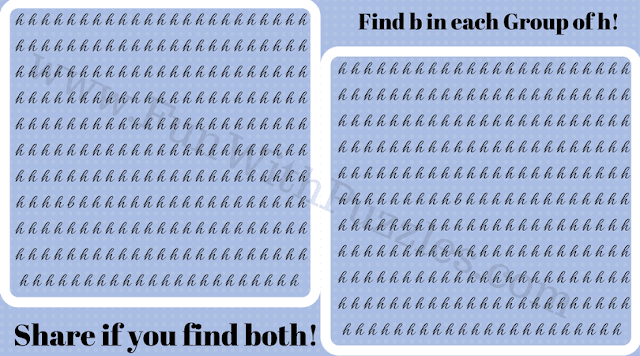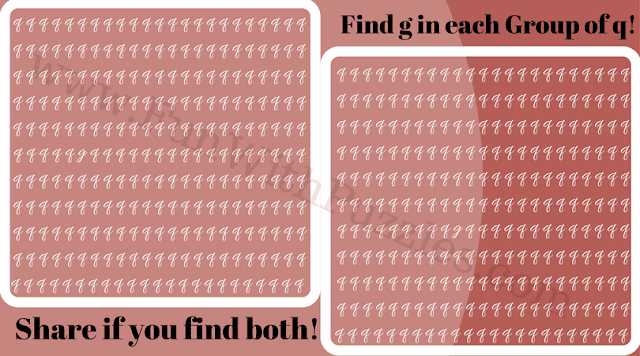Earlier we have published Crazy Puzzles to Test Your Eyesight. In these puzzles, one has to find the mentioned hidden letter in the given picture. The picture contains the two sets of different letters in which only one letter will be different. This is the challenge to find this given hidden letter. Today's Picture Puzzles to find hidden letters is the extension of these picture puzzles. Do first solve the Fun Puzzles to find C in given Patterns for Kids as these are easy puzzles, then come back to this post to solve these tough puzzles.
In these puzzles, there are pictures that contain two sections. In each section, a set of letters is provided. However, one letter is different in each of the sets from the letters in the set. This hidden letter is named at the top of each picture. Your challenge to find this hidden letter in each of these sections.
Do share this picture in case you are able to solve these puzzles. Answers link to these puzzles is given at the end of this post.1. Can You Find Hidden 'Y' in each group of X?2. Can You Find Hidden 'p' in each group of q?3. Can You Find Hidden 'b' in each group of h?4. Can You Find Hidden 'g' in each group of q?5. Can You Find Hidden 'i' in each group of t?